# Chapter 2: 3D Game Basics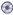Download CD Content

## The 3D Coordinate System

To begin our discussion of 3D basics, we ll first look at a one-dimensional (1D) world, which consists of the line that is shown in Figure 2.1.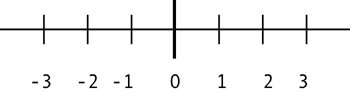Figure 2.1: The one-dimensional world.

When we say that the world consists of the line, we mean that in this world, it s only possible to move along this line. We ll call the line the x-axis.

If you were told to move to x = 2, you would know exactly where to go: to the spot marked 2 on the line. Likewise, if you were told to move to x = “3, you would also know exactly where to go. You ve probably already figured out that anything to the left of the point marked 0 (sometimes known as the origin ) has a negative value (with a minus ( “) sign), while anything to the right has a positive value (with a plus (+) sign, but we usually leave this sign out). You can see that the origin is at x = 0.

Pretty easy so far, huh? Well, it won t get much harder, but please pay attention to what happens next .

Now let s look at a two-dimensional (or 2D) world, where we add another axis. We will call the new axis the y-axis, as shown in Figure 2.2.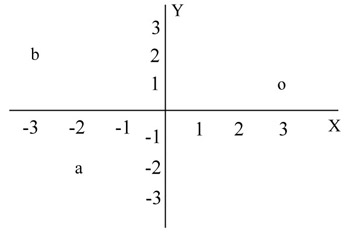Figure 2.2: A two-dimensional world.

If someone were to tell you to move to the spot x = 3, y = 1 you would know exactly where to go: about where the o is marked in Figure 2.2. Typically we would not say an object is at x = 3, y = 1, because this is a little cumbersome. Instead, we simplify this to say that the object is at (3, 1). By convention, the x coordinate is listed first, and the y coordinate is listed second.

Try two more to make sure you understand. The spot marked with the letter a in Figure 2.2 is at ( “2, “2), while b is at ( “3, 2).

Unlike early video games, modern games occur in a world like our own ”one that consists of three dimensions (3D). In the real world, the spot in Figure 2.2 marked o at (3, 1) could be on the paper you are looking at, or it could be two inches directly over the same spot. In other words, in addition to motion on the x and y axes, the third dimension allows motion along an axis that goes in and out of the paper. This third axis is called, reasonably enough, the z-axis, as shown in Figure 2.3.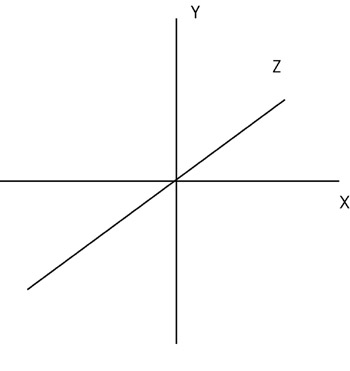Figure 2.3: A 3D world.

Typically, 3D worlds are drawn on paper using isometric views (meaning that it appears as if you were looking at the 3D figure from an angle). Figure 2.4 shows an isometric view of a cube, with the three axes.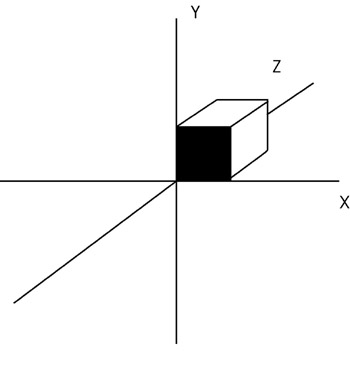Figure 2.4: An isometric view of a cube in a 3D world.

You will notice that the z-axis appears to be going into the paper, if the paper were viewed from the side. Figure 2.5 shows the positive and negative axes in the 3D world.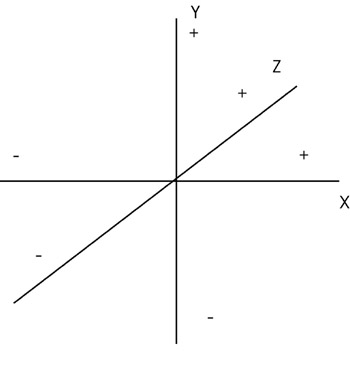Figure 2.5: Positive and negative axes in the 3D world.

You can now see that if you have an x, y, z coordinate system set up in your 3D world, you can exactly specify where an object is in your 3D world. For example, latitude and longitude tell you where you are on the surface of the earth (x and z coordinates), but to specify the location of an aircraft, you would also have to give its altitude (y coordinate).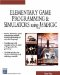Elementary Game Programming & Simulations Using Jamagic (Charles River Media Game Development)
ISBN: 1584502614
EAN: 2147483647
Year: 2002
Pages: 105
Authors: Sergio Perez